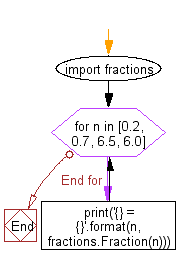﻿ Python Math: Create the fraction instances of float numbers - w3resource# Python Math: Create the fraction instances of float numbers

## Python Math: Exercise-44 with Solution

Write a Python program to create the fraction instances of float numbers.

Sample Solution:-

Python Code:

``````import fractions
for n in [0.2, 0.7, 6.5, 6.0]:
print('{} = {}'.format(n, fractions.Fraction(n)))
```
```

Sample Output:

```0.2 = 3602879701896397/18014398509481984

0.7 = 3152519739159347/4503599627370496

6.5 = 13/2

6.0 = 6
```

Flowchart:Python Code Editor:

Have another way to solve this solution? Contribute your code (and comments) through Disqus.

What is the difficulty level of this exercise?

Test your Programming skills with w3resource's quiz.

﻿

```>>> students = [{'name': 'John', 'score': 98}, {'name': 'Mike', 'score': 94}, {'name': 'Jennifer', 'score': 99}]